## 2.2.1 Heat Flux

It can be observed from various experiments that heat flows from the hotter to the colder side. Since the matter that is involved shows a statistical behavior at micro and nano-scale level in terms of the BROWNian molecular motion, the previous statement can be formulated as: The most probable consequence is that heat flows spontaneously from the hotter to the colder side by diffusion and relaxation mechanisms. This is exactly the definition of the second law of thermodynamics found in .

The time derivative of the heat can be expressed by FOURIER's and LAMBERT's2.12 law, which is equivalent to the STEFAN2.13-BOLTZMANN law for grey radiators. These laws consider the spatial temperature gradient plus the heat flux density due to the surface radiation to the ambient, respectively: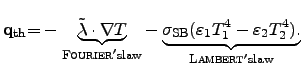(2.42)

Here, the first term on the right hand side is determined by FOURIER's law, where the thermal conductivity tensor is denoted by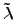and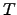is the local temperature in Kelvin. The second term describes LAMBERT's law for grey radiators, where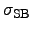denotes the STEFAN-BOLTZMANN constant and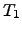and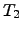stand for the ambient and the local surface temperature, respectively. The coefficients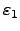and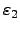reflect the efficiency of the absorption and the radiation of the considered surfaces. The black body'' has the absorption and radiation efficiency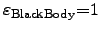. In most TCAD applications, the radiation can be neglected, except for areas at the surface of semiconductor devices, for instance, in passivation layers and heat sinks.

As the electric and magnetic fields store energy, also the matter stores heat energy. If heated bodies are put into a colder environment, they show a certain thermal relaxation behavior. A possible way to describe this relaxation behavior is to assign a quantity to each material, where the value of the quantity determines how much energy can be stored per mass or per mole. This quantity is called specific heat capacitance2.14. Historically, the heat capacitance is distinguished by two types. The first one determines the heat capacitance at constant pressure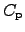and the second one describes the heat capacitance at constant volume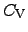: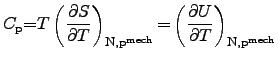(2.43)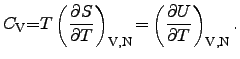(2.44)

Here, the heat capacitancesanddetermine the change of the internal energy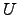with regard to the temperature change where different constraints are applied: constant pressure and constant volume. To obtain the specific heat capacitances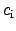, the heat capacitances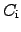are normalized to their involved mass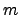: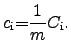(2.45)

The unit of the specific heat capacitance is either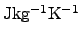or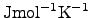according to the type of the mass used in (2.45) (mass or molar mass). The different values for the specific heat capacitances of a particular material can be easily transformed into each other.

Both heat capacitances of a given material, the one at constant pressure (cf. (2.43)) and the one at constant volume (cf. (2.44)), differ from each other by the identity(2.46)

where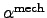denotes the thermal expansion coefficient at constant pressure, and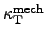represents the isothermal compressibility coefficient of the material . The thermal volume expansion coefficientand the compressibilitiesand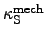are defined as follows: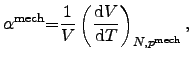(2.47)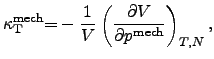(2.48)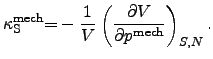(2.49)

Here, thedetermines the relative volume change with regard to temperature changes,shows the relative isothermal volume change andthe relative isentropic volume change with regard to changes of the local pressure.

Together with the thermodynamic potentials (2.31) and (2.32) and the thermodynamic identities (2.36) and (2.37), another correlation between heat capacitances and compressibilities can be derived by using the chain rule for differentiation.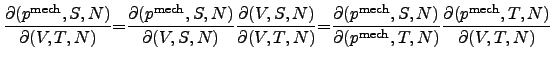(2.50)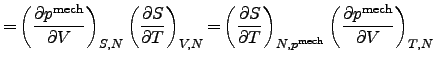(2.51)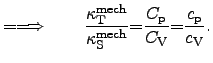(2.52)

The equations (2.50) and (2.51) show the equality of the different equivalent methods for differentiation according to the chain rules from LEIBNIZ2.15 and with the previous definitions of the compressibilities (2.48) and (2.49). Since the isobar and isochor heat capacitances describe the same region of matter, their ratio in (2.51) is the same as for the corresponding specific heat capacitances.

For isotropic and temperature-independent materials, the left hand side of (2.9) becomes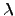times the LAPLACEian2.16operator and (2.9) can be written as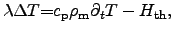(2.53)

where the maximum of the thermal conductivity has been published for carbo-nano-tubes (CNTs) and nano wires as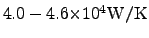in [77,78]. In comparison to that, the thermal conductivity of diamond is typically in the range of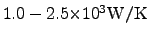[79,80].

To determine the proper heat generation term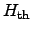for a particular problem, several proposals have been made for semiconductor and interconnect models. The simplest model is to calculate the power loss with the local electrical field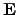and the resulting local current density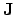[81,82] by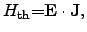(2.54)

whereandcan be calculated using the appropriate models to describe the observed behavior of the electrical field and the electrical current density. In order to account for the current densities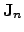and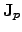appropriately, the SEEBECK2.17 effect has to be considered as well, where the phenomenological semiconductor current equations [62,66] can be enhanced by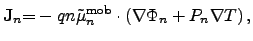(2.55)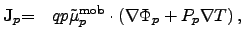(2.56)

where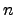and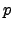represent the carrier concentrations for electrons and holes,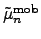and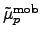denote the mobility tensors for electrons and holes, and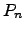and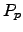are the SEEBECK coefficients for electrons and holes. The quantities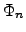and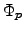represent the quasi-FERMI2.18 potentials for electrons and holes in semiconductor materials: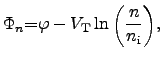(2.57)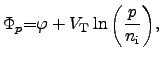(2.58)

where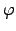denotes the local potential,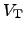is the thermal voltage according to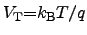, and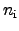denotes the intrinsic carrier concentration of the semiconductor material.

For semiconductor devices, the temperatureis often assumed to be the lattice temperature of the semiconductor crystal since the carriers and the lattice can be considered as two systems in thermal quasi equilibrium . For a rigorous treatment of the SEEBECK effect, also the FOURIER law for the heat conduction equation (2.8) has to be adapted to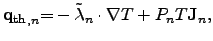(2.59)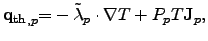(2.60)

where the first part is due to FOURIER's law and the second part due to SEEBECK's effect.

Stefan Holzer 2007-11-19